# Math Practice Topic: Identifying Angles

 Description: Given a figure, classify angles as vertical, adjacent, complementary, supplementary, and/or corresponding. Adaptive Learning Progression: The number of intersecting lines increases. Start using MathScore for free

## Sample Levels (out of 4)

Classify the following angles that are labeled.

 1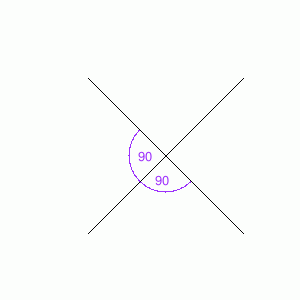Vertical Angles        Adjacent Angles        Complementary Angles        Supplementary Angles        Corresponding Angles        None of the above 2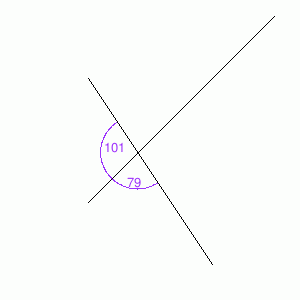Vertical Angles        Adjacent Angles        Complementary Angles        Supplementary Angles        Corresponding Angles        None of the above

Classify the following angles that are labeled.

 1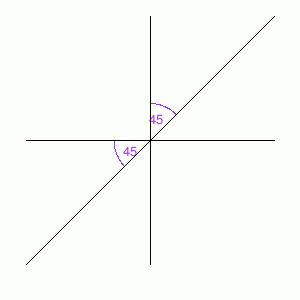Vertical Angles        Adjacent Angles        Complementary Angles        Supplementary Angles        Corresponding Angles        None of the above 2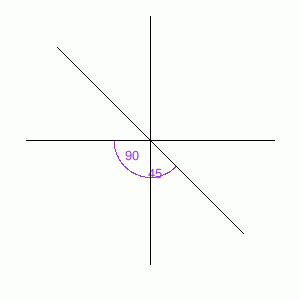Vertical Angles        Adjacent Angles        Complementary Angles        Supplementary Angles        Corresponding Angles        None of the above

Classify the following angles that are labeled.

 1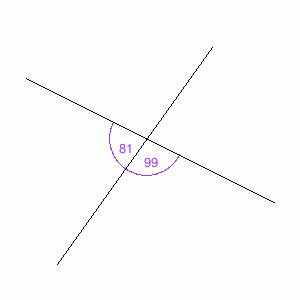Vertical Angles        Adjacent Angles        Complementary Angles        Supplementary Angles        Corresponding Angles        None of the above 2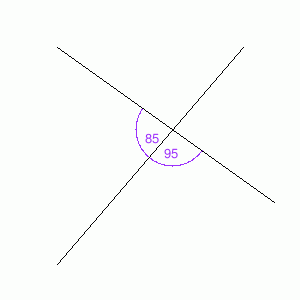Vertical Angles        Adjacent Angles        Complementary Angles        Supplementary Angles        Corresponding Angles        None of the above